# Civil Engineering - Theory of Structures

### Exercise :: Theory of Structures - Section 3

11.

A composite beam is composed of two equal strips one of brass and other of steel. If the temperature is raised

 A. steel experiences tensile force B. brass experiences compressive force C. composite beam gets subjected to a couple D. composite beam bends E. All the above.

Explanation:

No answer description available for this question. Let us discuss.

12.

A two hinged parabolic arch of span l and rise h carries a load varying from zero at the left end to ω per unit run at the right end. The horizontal thrust is

 A.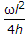B.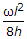C.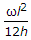D.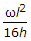Explanation:

No answer description available for this question. Let us discuss.

13.

The force in EC of the truss shown in the given figure, is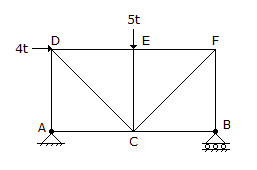A. zero B. 5t tension C. 5t compression D. 4t tension E. None of these.

Explanation:

No answer description available for this question. Let us discuss.

14.

Shear centre of a half circular section of radius r and of constant thickness, lies at a distance of x from the centre where x is

 A.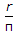B.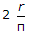C.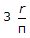D.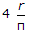Explanation:

No answer description available for this question. Let us discuss.

15.

Pick up the correct statement from the following:

 A. The moment of inertia is calculated about the axis about which bending takes place B. If tensile stress is less than axial stress, the section experiences compressive stress C. If tensile stress is equal to axial stress, the section experiences compressive stress D. If tensile stress is more than axial stress, some portion of the section experiences a tensile stress E. All the above.